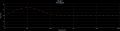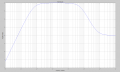# capacitor as short and open - calculate gain and freq.

#### johnyjj2

Joined Dec 11, 2009
7
Hello!

I've got exercise in which I need to determine values of gain k and frequencies f, which are shown on the plot. For the simple graph I know when to replace capacitor with short, open or not to replace. However, I don't know how to replace those for more difficult graphs.

In the case of graph decreasing for higher frequencies, I replace capacitors in such a manner:
what I want to calculate - first capacitor - second capacitor
k1 - open - open
f1 - capacitor - open
k2 - short - open
f2 - short - capacitor
k3 - short - short

What to do in the case of other graphs?

Main question and graphs: http://images47.fotosik.pl/306/5c69f37386f470a1.jpg

Regards!

Last edited:

#### Georacer

Joined Nov 25, 2009
5,182
It is not as obvious as the previous circuit how the capacitors behave in the various frequencies. Forming the transfer function of the inverting amplifier (T(s)=-Z2/Z1) will hint you about the response of the circuit in relation to the frequency. Try to calculate the transfer function without substituting values, and your exercise will become a lot easier.
So, extract the transfer function and we'll talk more about it.

I hope I pointed you to the right direction.

#### johnyjj2

Joined Dec 11, 2009
7

You mean to calculate gain for the circuit with capacitors as 1/jwC, without any shorts or opens? If yes, I'm going to calculate it tomorrow.

Regards!

#### Georacer

Joined Nov 25, 2009
5,182
I tried to solve the exercise myself and I was in for a surprise! It turned out that forming the tf was easy, but solving it for every unknown was a pain, as it contained variables in squares... under roots... on denominators!!!
So I thought "there's got to be an easier way..." and that got me to see what the exercise really wanted us to do, and the beauty of it.

As I don't want to spoil the pleasure of coming to the solution yourself, I will give you an outline of the solution.

• Form the tf by using the variables of resistanses and capacitors
• Factor it in a way that it's a product of two tf's. The first is an RC-tf with a time constant of R1C1. The second is a sum of a constant and an RC-tf with time constant of R2C2
• Now, theory tells us that the product of tf's is an overlay of the two tf. That means that if we put a HP and a LP filter one after another, the tf of the new system is the product of the two former.
• Using this, use the data on the plot to isolate each filter on an area of the plot and calculate its characteristic values (time constant, gain). Hint: start with the HP filter.
• Crunch the numbers while you solve some really simple equations and, voila, you got your values. Note that the gain of a RC-tf must be converted to dB's in order to compare it with the one on the graph.
I simulated the circuit with the values I found and came to this plot: It's ok in the low freqs, but I can't understand it's loss of gain so soon in the x-axis. I might as well check my numbers...#### Georacer

Joined Nov 25, 2009
5,182
My calculations were correct. As I didn't like the previous bode plot, I extracted the tf and gave it to Matlab. I ended up with something like this:It's better than the previous one but still off by a decade from the expected. I can't say I' an expert in circuit analysis, so I hope this is an expected error stemming from the fact that we analysed a theoretical filter graph and tried to extract data from it.
It was good fun messing with the plot though!#### t_n_k

Joined Mar 6, 2009
5,455
For your second problem, the three gains will come out based on the following assumptions.

K1 - C1 & C2 are open circuit
K2 - C1 is a short circuit and C2 is open circuit
K3 - C1 & C2 are both short circuit.

To find K1

Gain K1 = [R3+R5+R6]/R6 = 100k/1k=100

#### johnyjj2

Joined Dec 11, 2009
7
Thank you for your answers!

Georacer: I used for [the other task] formula for inverting amplifier and found that K(s) = - K1(s) * K2(s), where K1(s) = [jwC1] / [R1jwC1 + 1] = 40 dB and K2(s) = [R3 * (1 + R2jwC2) + R2] / [1 + R2jwC2] = 20 dB. However, I don't know how to distinguish between HP and LP and how to use data from plot. And yeah, I'm sure those exercises can be exciting but I still lack some knowledge.

t_n_k: What you gave is solution for [one task], which is also given in my first post as [main question and graphs]. However, I still wonder how I can benefit from those plots, what are general rules when capacitors are replaced by short, open or without replacement, how to proceed with this kind of exercises because as Georacer noticed for one of those tasks there are at least two ways, exhaustive and simple one.

Regards!

#### Georacer

Joined Nov 25, 2009
5,182
First let us find the tf in the s-domain: as you noticed it is:
$$T(s)=-\frac{s\cdot{C1}}{1+s\cdot{C1}\cdot{R1}}\cdot\left(R3+\frac{R2}{1+s\cdot{C2}\cdot{R2}}\right)$$

Now, you know that a tf of the form
$$Th(s)=\frac{K}{1+s\cdot\tau}$$
is a LP filter with a time constant of τ (we will assume τ=R*C for this exercise, as it is a simple RC filter)

On the contrary
$$Tl(s)=\frac{s\cdot{K}\cdot\tau}{1+s\tau}$$
is a HP filter.

It is now clear how our tf is an overlay of a HP filter wiht an LP filter with a steady AC gain (represented by the R3). Pay attention: in T(s) the first fraction is the HP filter and the parenthesis (wich contains the second fraction) is the LP filter.

In the frequencies from 10Hz to 10kHz the HP behaves as its capacitors were shorted, whereas the LP filter has its capacitors open. That allows us to substitue s with infinite in the HP fraction, and s with zero in the parenthesis. The resulting product is the gain k1, as an expression of R1,R2,C2 and R3. ATTENTION! We have found k1 in v/v not dB's and must convert it before saying it is equal with 20dB. (k(dB)=20log(K(s)))

In the frequences from 10kHz and forth, both filters' capacitors behave as shorts and that allows us to substitute s with infinite for the whole formula. The resulting product is the gain k2, as an expression of R1 and R3

Now, let's make sure we know a little bit of theory before going on. Notice the spots where the graph "breaks" and forms corners. These are called threshold frequencies. The first corresponds to the HP filter and the second to the LP. This frequency has a formula: $$f_\small{0}=\frac1{2\cdot\pi\τ}\( As a result we can now equate the threshold frequency of the HP with 10Hz. This lets us calculate R1. Do the same with the threshold frequency of 10kHz using the time constant of the LP and you can find a relation between R2 and C2. That's it! You now have enough equations to solve your problem. Few! That took a while! I hope now you can fight your way through.$$\)

Last edited:

#### t_n_k

Joined Mar 6, 2009
5,455
t_n_k: What you gave is solution for [one task], which is also given in my first post as [main question and graphs]. However, I still wonder how I can benefit from those plots, what are general rules when capacitors are replaced by short, open or without replacement, how to proceed with this kind of exercises because as Georacer noticed for one of those tasks there are at least two ways, exhaustive and simple one.
Exhaustive - it takes a lot of time to get to a more accurate solution.

Simple - some approximations are made to get to a quicker solution.

Depends on the purpose of the analysis. Do you need an accurate analysis or a only reasonable approximation to give you an idea on how to proceed with a design or to gain an understanding of the overall circuit function?

The substitution of caps with a short or open circuit on so on is clearly an approximation approach.

With the plethora of circuit simulation software available you also have a third option, but one which can make the user overly reliant (dependent?) on those tools or unwary of the pitfalls they might encounter in applying them indiscriminately. Also, it's paramount for the student's confidence and skill development to be able to perform and verify a detailed analytical solution - now and again.